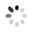# |贴子

2015-09-06
设x、y、z是两两不同的实数，且满足y=x(4-x)①z=y(4-y)②x=z(4-z)③求x+y+z所有可能值 要...
2015-09-06
设x、y、z是两两不同的实数，且满足y=x(4-x)①z=y(4-y)②x=z(4-z)③求x+y+z所有可能值 要...
2015-09-06
设x、y、z是两两不同的实数，且满足y=x(4-x)①z=y(4-y)②x=z(4-z)③求x+y+z所有可能值
2015-09-06
设x、y、z是两两不同的实数，且满足y=x(4-x)①z=y(4-y)②x=z(4-z)③求x+y+z所有可能值
2015-09-06
设x、y、z是两两不同的实数，且满足y=x(4-x)①z=y(4-y)②x=z(4-z)③求x+y+z所有可能值
2015-09-06
设x、y、z是两两不同的实数，且满足y=x(4-x)①z=y(4-y)②x=z(4-z)③求x+y+z所有可能值TA还没有收到任何礼物~